dummy

# 基本概念

## 程序语言的语法描述

### 基本概念

• 字母表

• 符号串

• 符号串集合

• 符号串的运算

• 相等

• 长度

• 就是两个连起来，且连起来的积也是$$\Sigma$$上的符号串

• 符号串集合的运算

• 乘积

按顺序枚举连接即可得到，如A={s,t},B={u,v} AB={su,sv,tu,tv}

• 幂运算

就按乘法来就可以

• 闭包

$$A^+=A^1 \cup A^2 \cup ... \cup A^n$$为正闭包

$$A^* = A^0 \cup A^+$$为A的闭包

### 约定

• 非终结符

A,B,C

• 终结符

a,b,c

• 终结符/非终结符

X,Y,Z

• 终结符号串

x,y,z

• 终结符/非终结符构成的符号串

$$\alpha,\beta$$

• 在产生式A->$$\alpha$$

A是产生式的左边

$$\alpha$$是产生式的右边

### 文法

• $$V_N$$为非终结符号集
• $$V_T$$为终结符号集
• P为产生式（规则）集合
• S称作开始符号，至少要在一条规则中作为左部出现

# 词法分析

## 词法分析器

1. <$WHILE, _> 2. <$LPAR, _>

3. <$ID, pointer to i> 4. <$EQUAL, _>

5. <\$ID, pointer to j>

....

### 主要功能

• 读取源程序生成单词符号
• 过滤注释和空白
• 关联编译器生成的错误与源程序的位置。

## 状态转换图

• 结点代表状态
• 状态之间用箭弧连接
• 箭弧上的标记字符代表起始结点状态下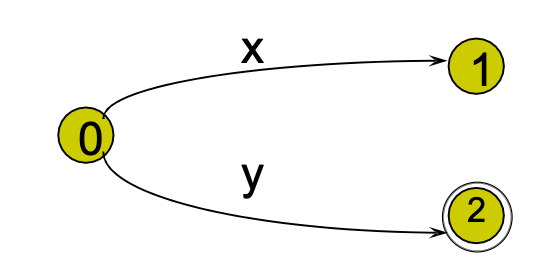image-20210315142248976

## 正则表达式和有限自动机

### 正则表达式和正则集

1. $$\epsilon$$$$\varnothing$$$$\Sigma$$上的正则式，其正则集为{$$\epsilon$$}和$$\varnothing$$
2. 任何$$a\in \Sigma$$，a是$$\Sigma$$上的一个正则式，正则集为{a}
3. 假定U和V都是$$\Sigma$$上的正则式，正则集为L(U)和L(V)，那么对UV的交并闭包，也能产生正则集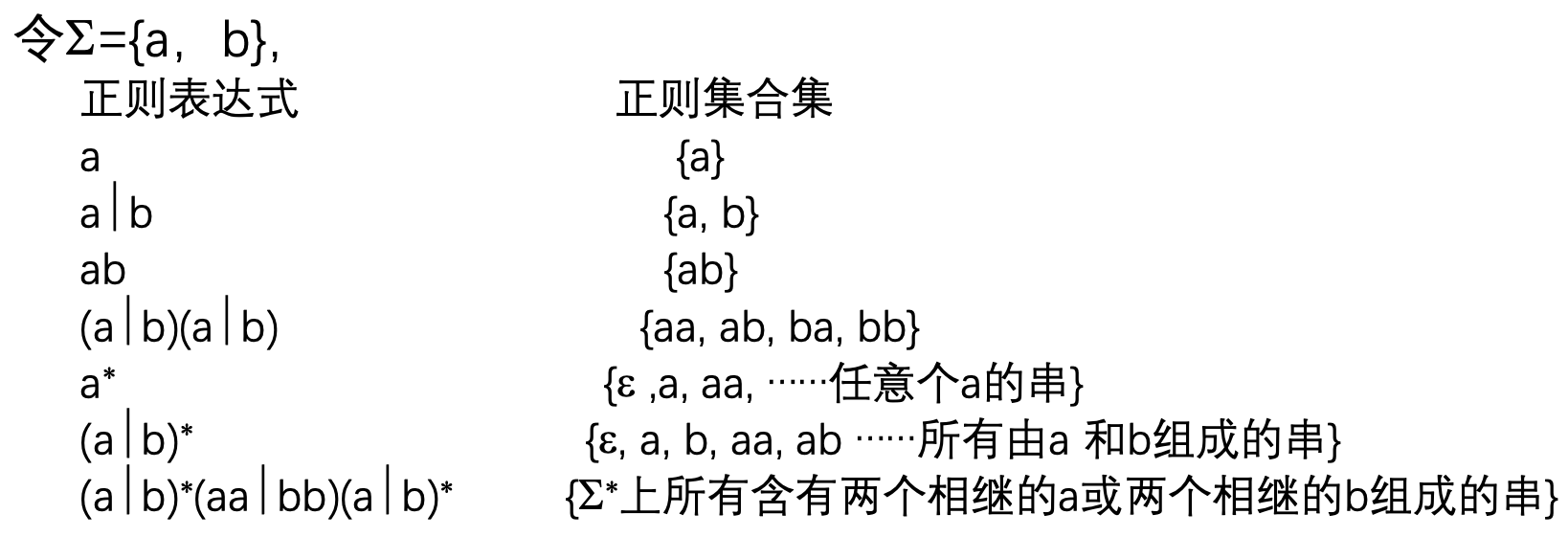image-20210315145818981

### 有限自动机

#### NFA的确定化

• $$\epsilon$$-闭包，定义为一状态集，包括
• I中的任何状态s
• I中s经过任意条$$\epsilon$$能够到达的状态的集合
• a弧转换Ia=$$\epsilon$$-closure(move(I, a)) = $$\epsilon$$-closure(J)，J定义为可从I的某一状态经过一条a弧而到达的状态的全体.

#### DFA最小化

• 不存在多余状态
• 不存在相互等价的两个状态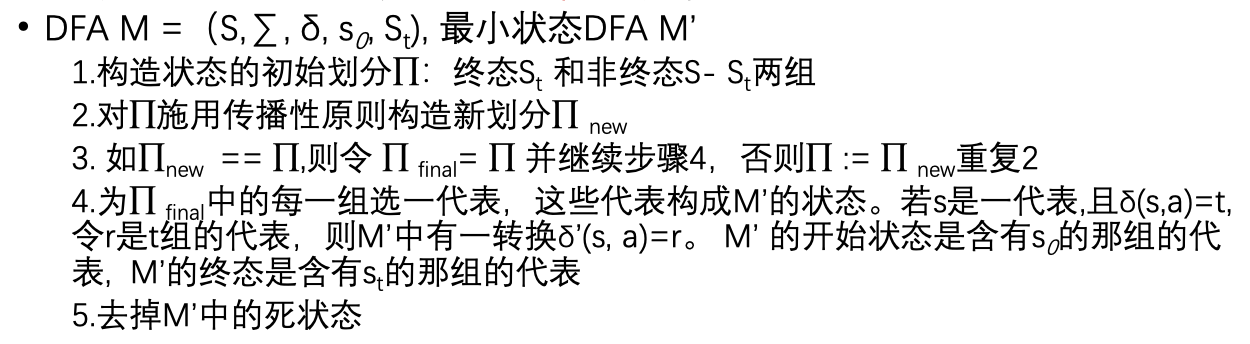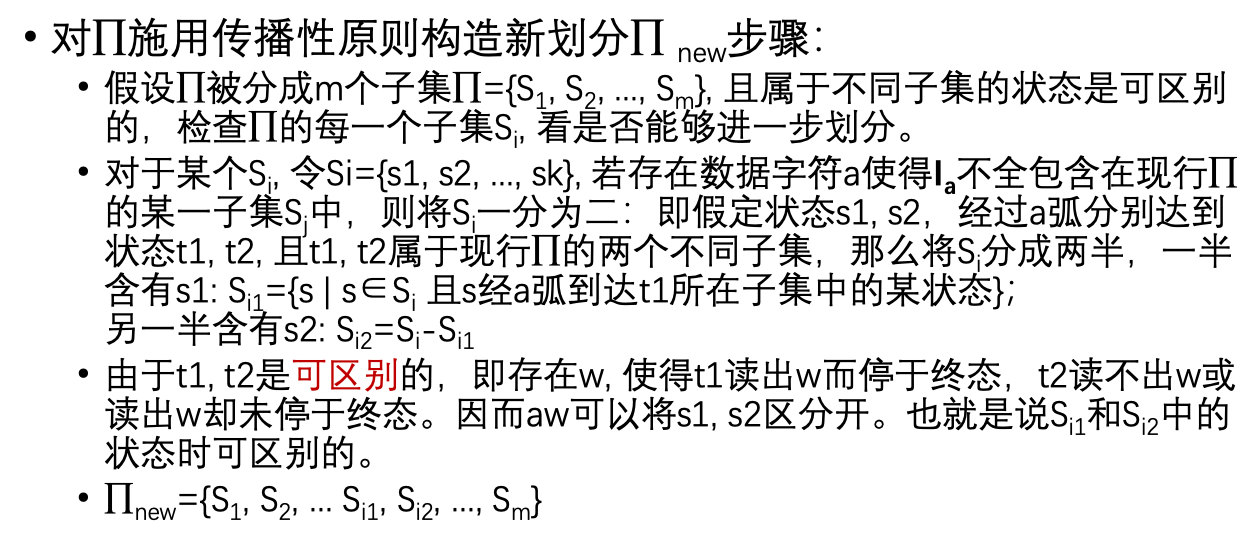## 词法分析程序的自动生成

LEX程序分为两部分：正则定义式为（简名->正则式）的组合，以及识别规则。即正则定义式和其识别出来后的动作。

LEX的工作过程就是将LEX源程序编译成一个.c文件，再通过C编译器编译成一个词法分析器。

# 自顶向下的语法分析

## LL(1)分析法

LL(1)中的第一个L表示从左到右扫描输入串，第二个L表示最左推导，1表示分析时每一步只需向前查看一个符号。

• 不含左递归
• 非终结符的产生式的FIRST集之间没有交集
• 对于每个非终结符，如果存在某个FIRST集包含$$\epsilon$$则FIRST和FOLLOW集没有交集

### 改造成ll1文法

#### 消除左递归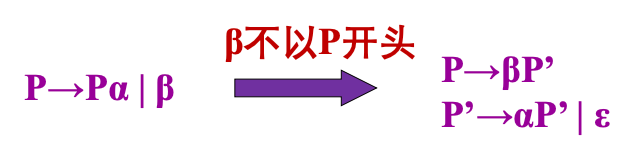image-20210322153137185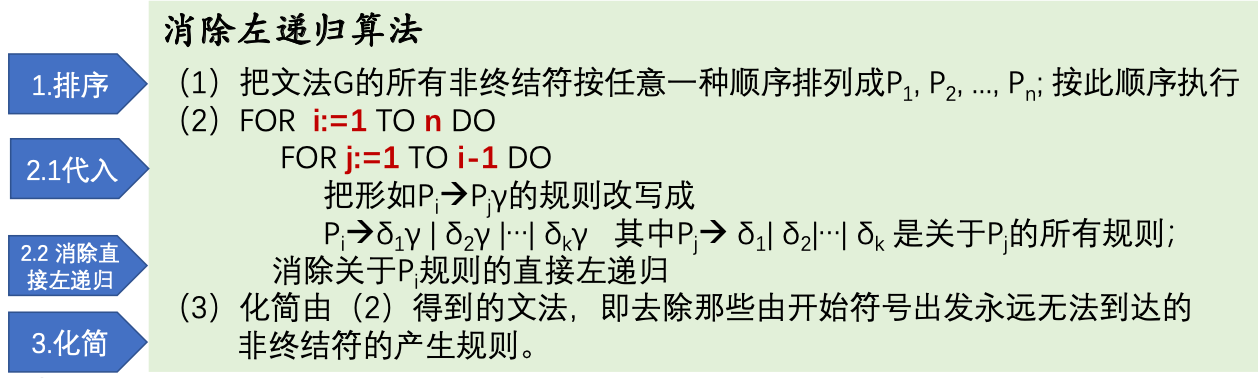image-20210322154143978

#### 消除回溯、提取左因子

FIRST和FOLLOW的推断可以看这个https://www.gatevidyalay.com/first-and-follow-compiler-design/

## 预测分析程序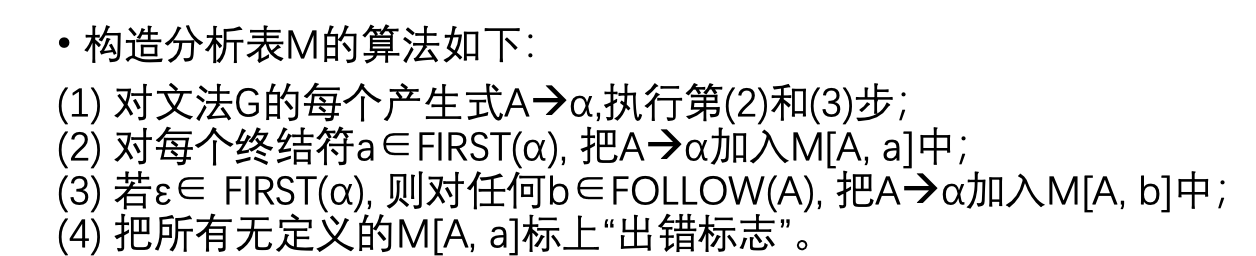image-20210329153500372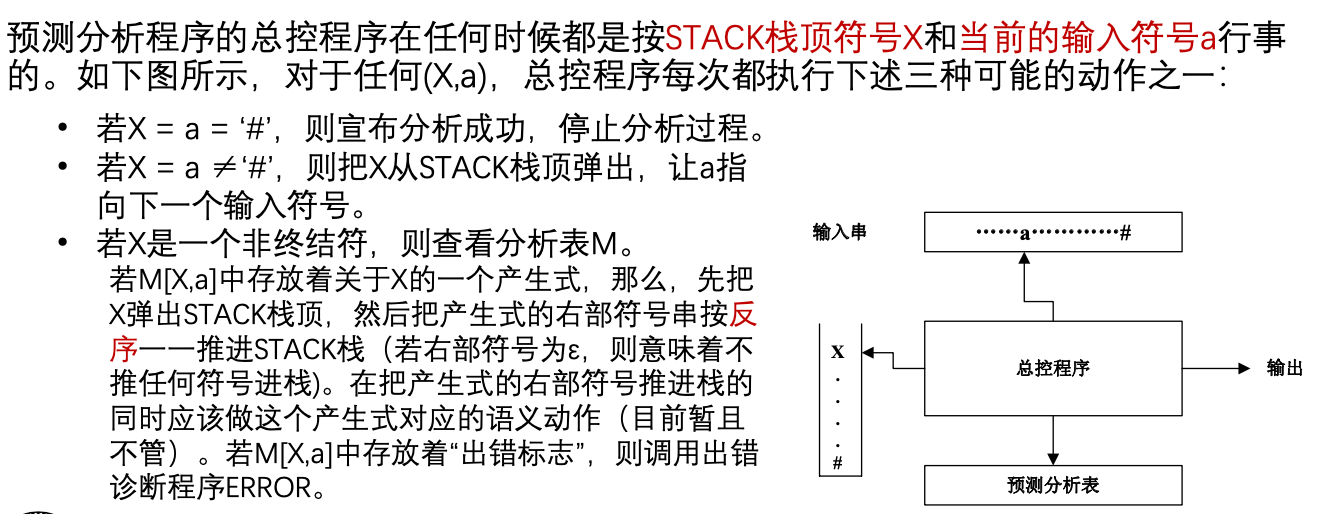image-20210329153623426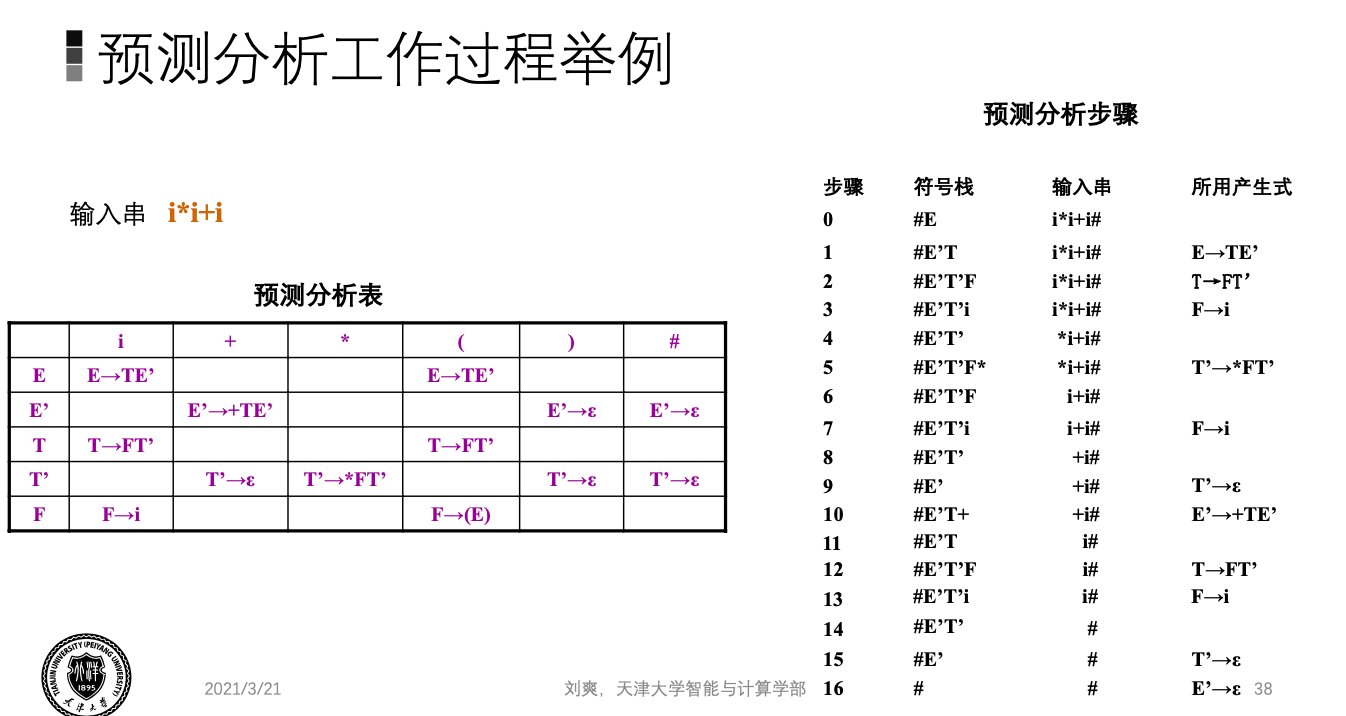image-20210329153958974

# 自下而上的语法分析

## 移动归约法

### 短语、直接短语、句柄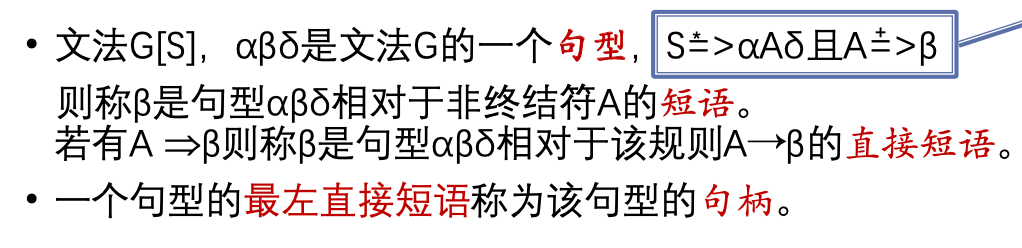image-20210329154922700

### 规范归约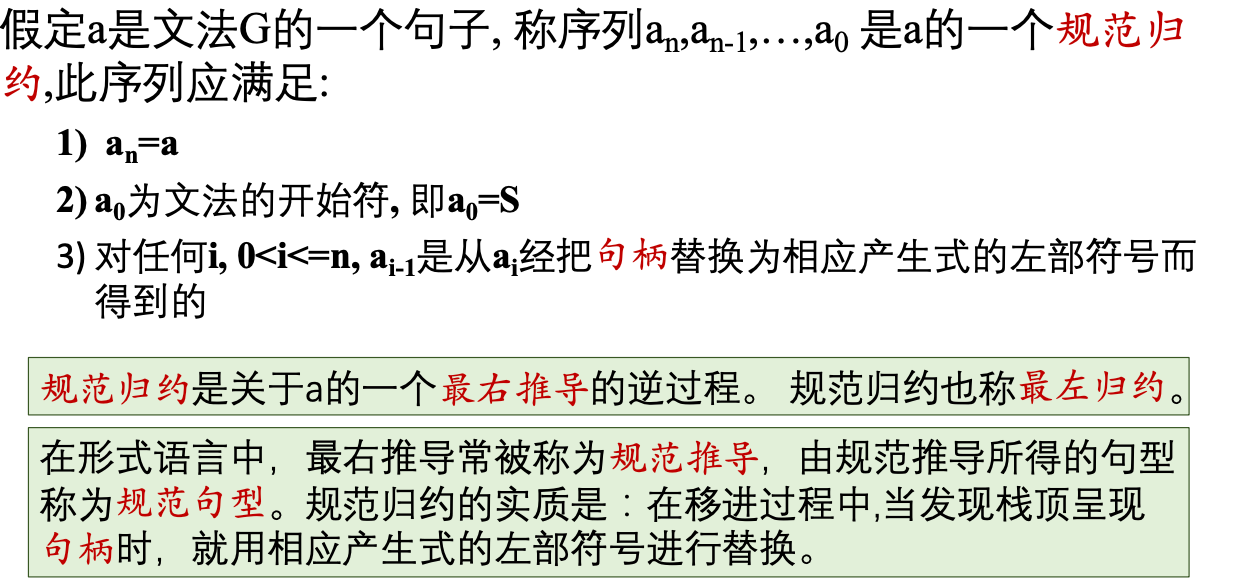image-20210329160816870

### 例子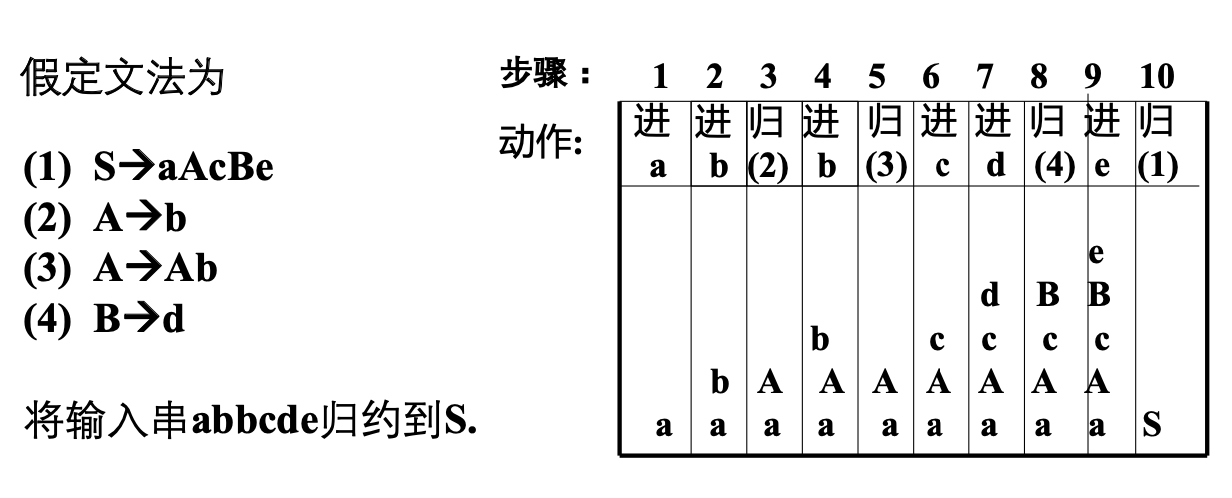image-20210331103209586

## 算符优先分析法

### 算法文法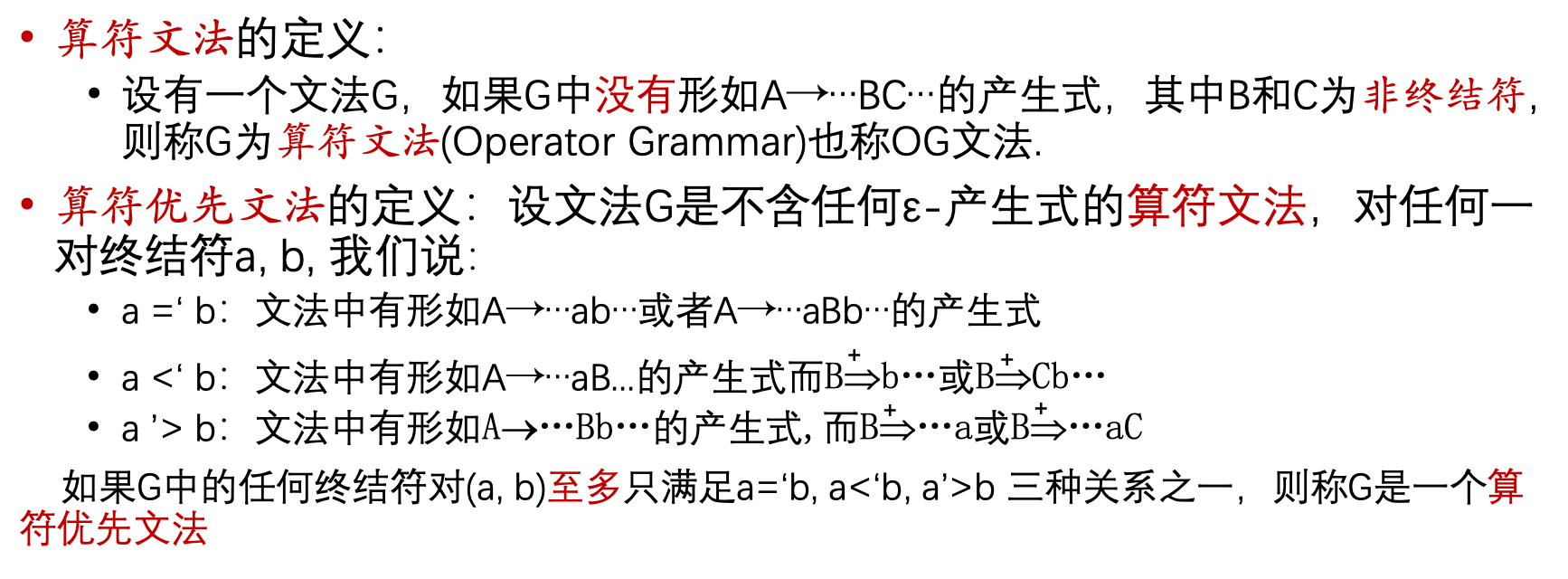image-20210331104404298

a=b，则a和b在同一个句柄中

a>`b，则a先被归约

### 素短语

$\#N_1a_1N_2a_2...N_na_nN_{a+1}\#$

### FIRSTVT LASTVT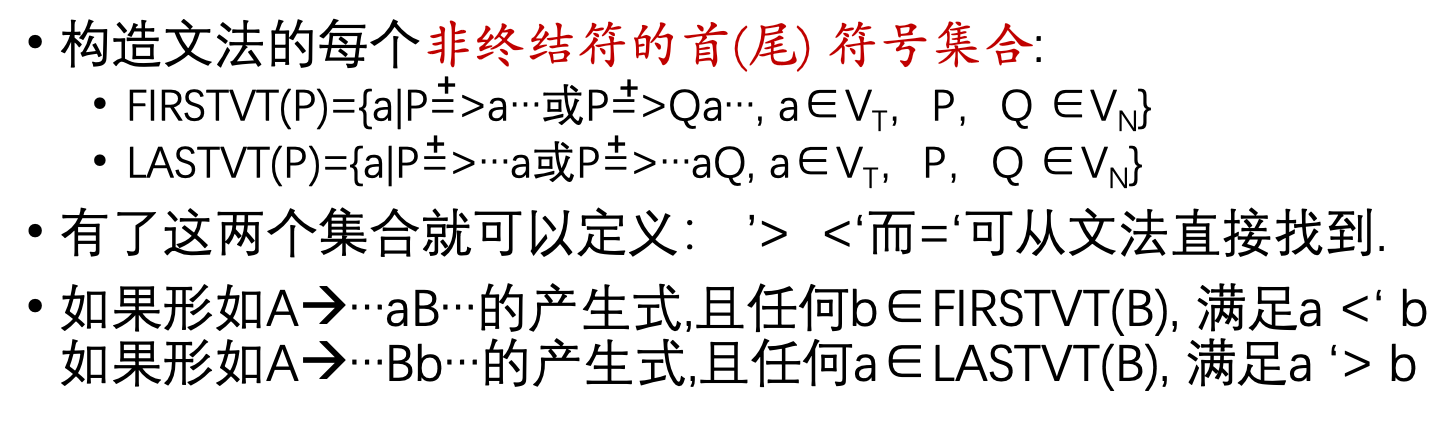image-20210412222914196

1. 若有P->a，或P->Qa，则将a加入集合
2. 若有a在Q的FIRSTVT集合中，又有P->Q，则a也在P的FIRSTVT中。

### 算法思想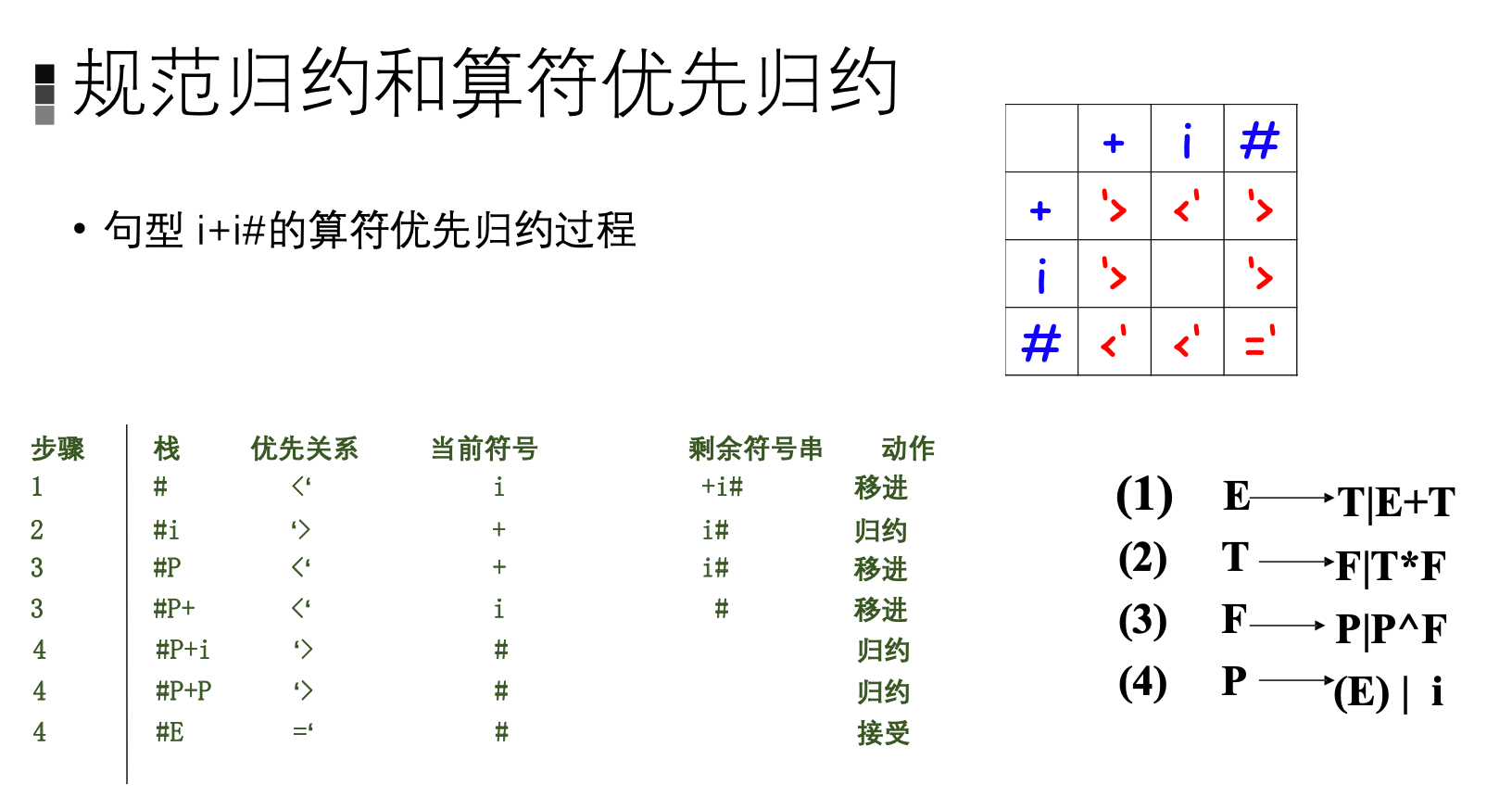image-20210412232128314

## LR分析法

LR分析的优点有以下几点:

• LR语法分析器能识别几乎所有能用上下文无关文法描述的程序设计语言的结构。

• LR分析法是已知的最一般的无回溯移动归约语法分析法，而且可以和其他移动 归约分析一样被有效地实现。

• LR分析法分析的文法类是预测分析法能分析的文法类的真超集。

• 在自左向右扫描输入符号串时，LR语法分析器能及时发现语法错误。

LR分析法的基本思想是，在规范归约过程中，一方面记住过去已经移进和归约出的整个符号串，另一方面用产生式推测未来可能遇到的输入符号。

### LR分析器结构

ACTION表

ACTION表中对应的动作有4种，分别是移进、归约、接收和报错。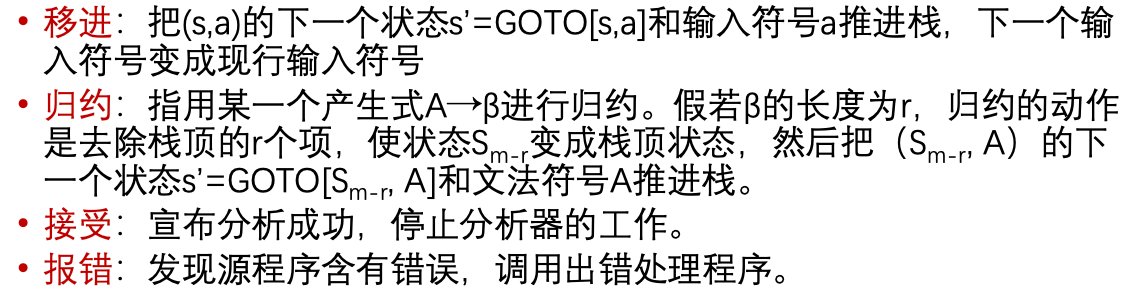image-20210419144408429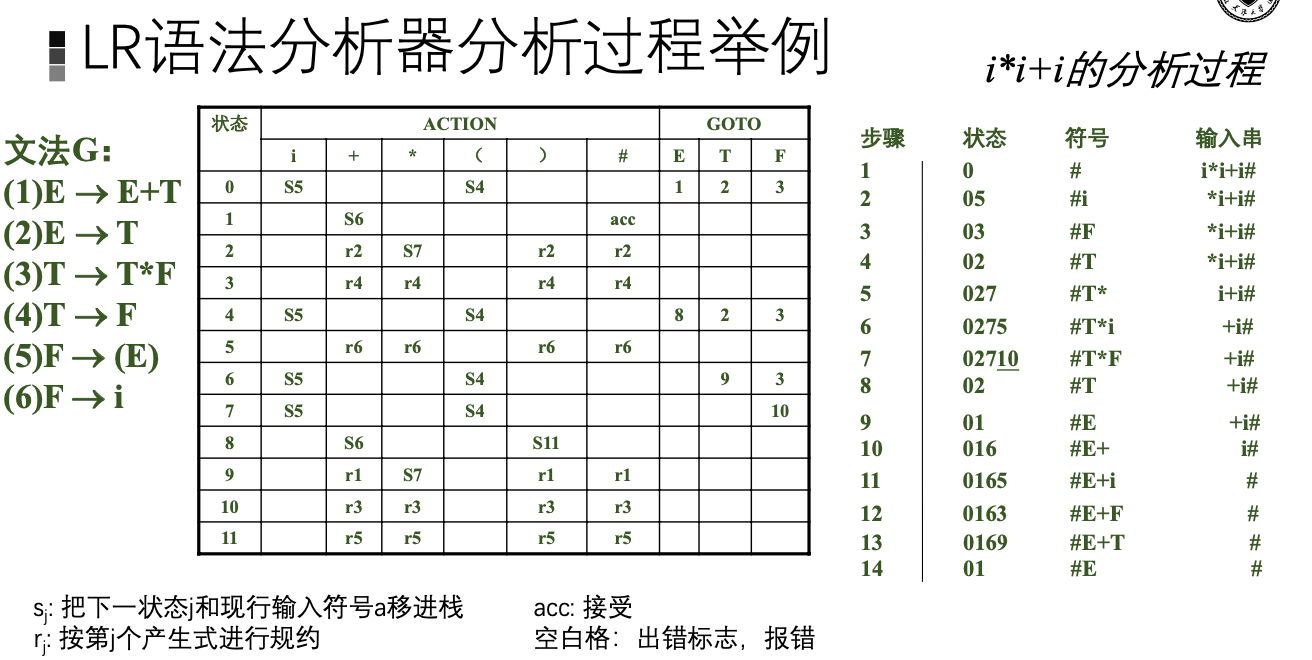image-20210419144506814

### LR(0)项目集族的构造

• 归约项目

• 接受项目

• 移进项目

• 待约项目

1. 将I中所有项目加入集合
2. 对任一·后是非终结符B的，将非终极符B在左边的产生式的项目B->·y加入集合
3. 重复12步直到无法增大

GO函数

GO函数在构造项目集规范族中起作用。

$$I_0$$中，假设有项目A->·aB，则可以令G($$I_0$$,a)=$$I_1$$为一个转化函数并且$$I_1$$就是CLOSUER({A->a·B})

### 分析表的构造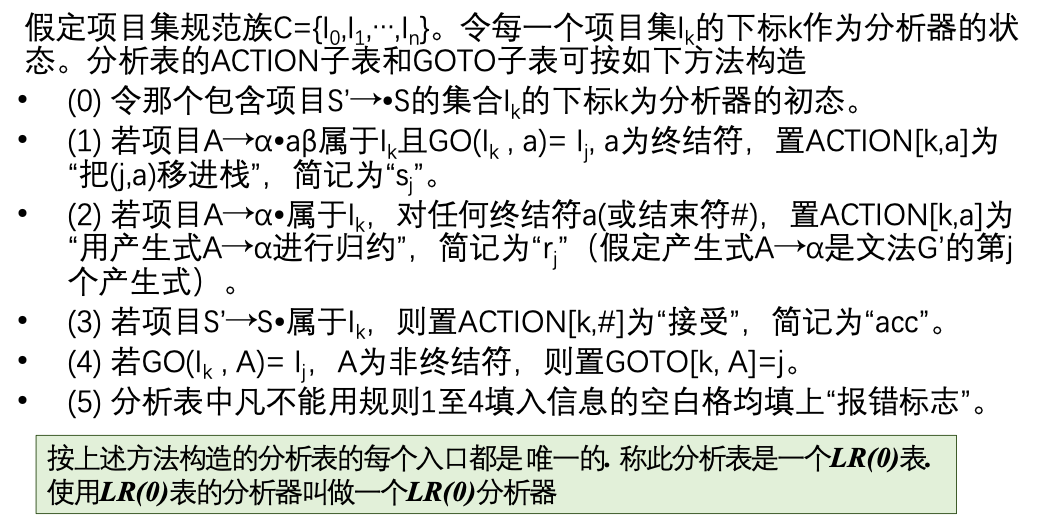image-20210419185255121

• 含移进项目和归约项目
• 含有多个归约项目

## SLR分析法

SLR利用了非终结符的FOLLOW集来解决冲突。

### SLR语法分析表的构造算法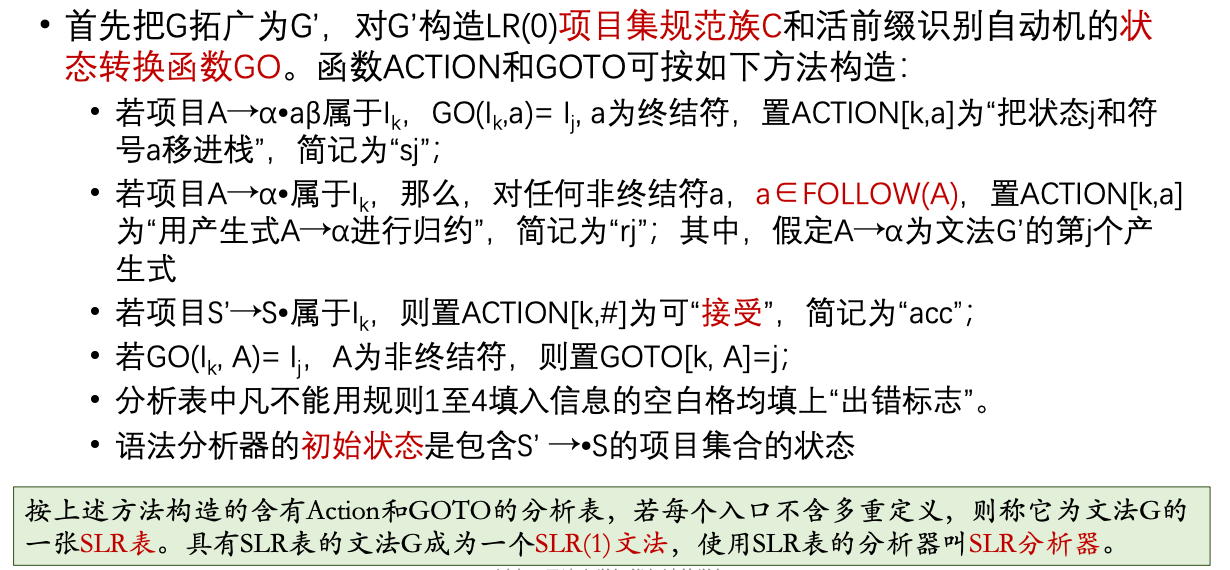image-20210419185327609

## 规范LR语法

CLOSURE闭包

• γ=δα

• a是ω的第一个符号，或者ω是空串，a是#。

• I的任何项目都属于CLOSURE(I)

• LR(0)项目的选取类似，向前搜索串定义为:

• 重复上面两步

### 语法分析表构造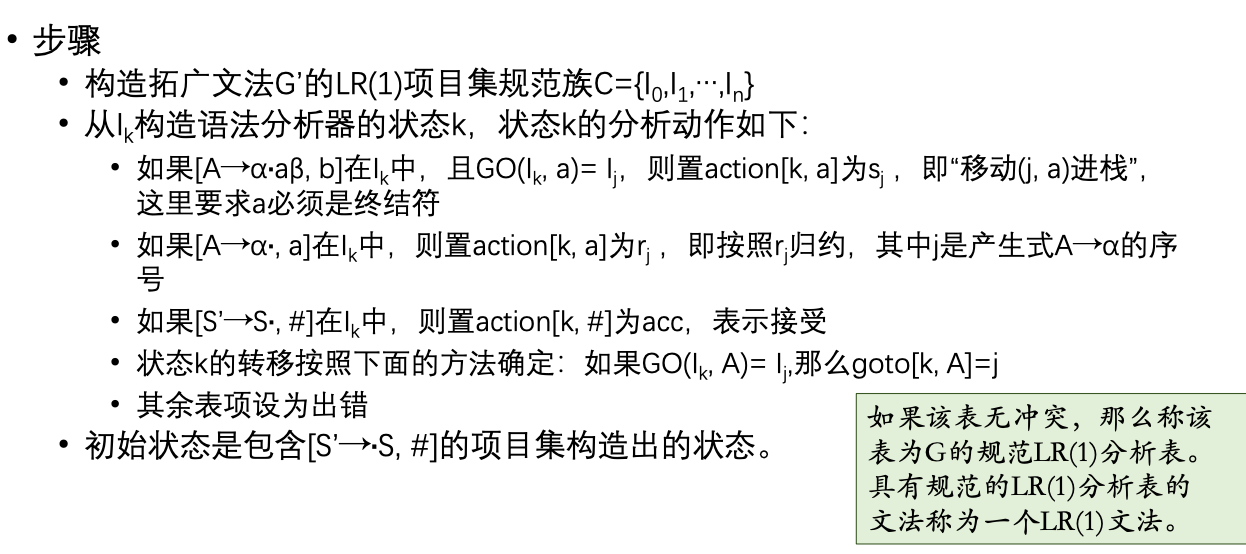image-20210420200147373

# 语义分析

## L属性文法的自上而下翻译

### 语法制导翻译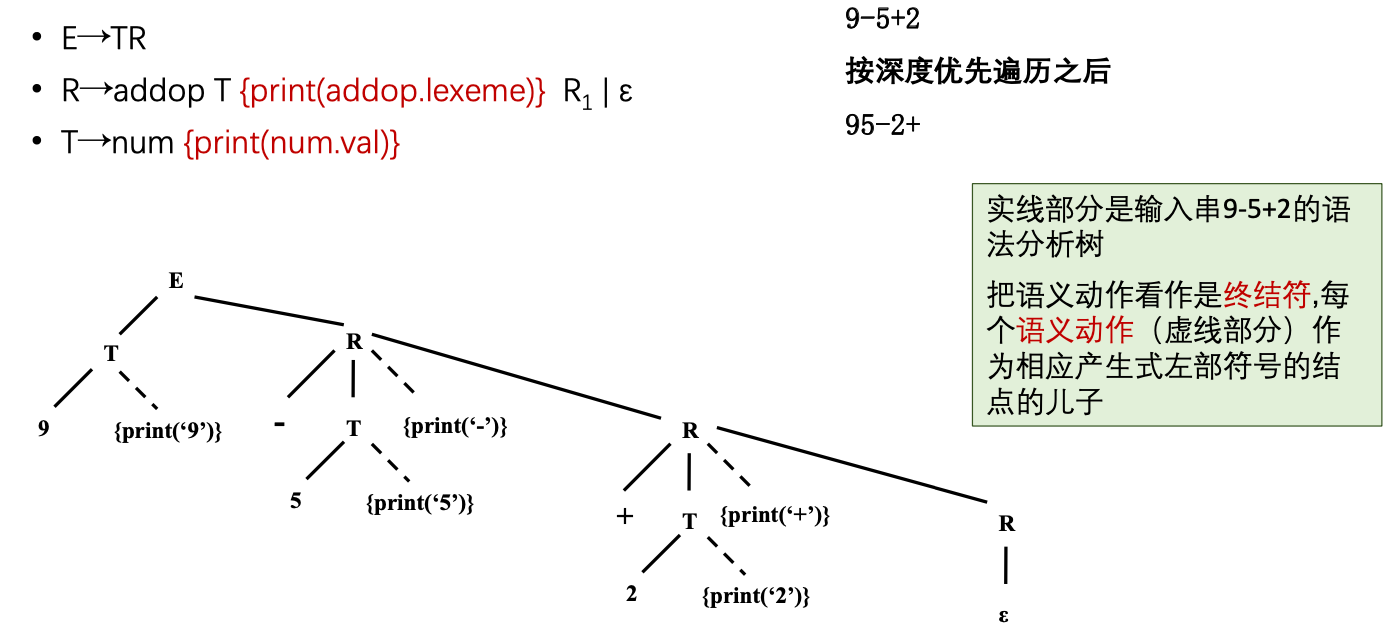image-20210421104256138

• (最基本)当动作引用某个属性时，这个属性必须是可用的。
• 只有综合属性时，为每一个语义规则建立一个包含赋值的动作，并且把动作放在末尾
• 同时存在综合属性和继承属性时
1. 产生式右部符号的继承属性必须在这个符号以前的动作中计算出来
2. 一个动作不能引用右部符号的综合属性
3. 产生式左部非终结符的综合属性只有在其引用的所有属性值都计算出来以后才能计算。计算该属性的动作通常放在产生式右部的末尾。